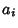## Constant Problem

Given an expression involving known constants, integration in finite terms, computation of limits, etc., determine if the expression is equal to Zero. The constant problem is a very difficult unsolved problem in Transcendental Number theory. However, it is known that the problem is Undecidable if the expression involves oscillatory functions such as Sine. However, the Ferguson-Forcade Algorithm is a practical algorithm for determining if there exist integersfor given real numberssuch thator else establish bounds within which no relation can exist (Bailey 1988).

Bailey, D. H. Numerical Results on the Transcendence of Constants Involving,, and Euler's Constant.'' Math. Comput. 50, 275-281, 1988.
Sackell, J. Zero-Equivalence in Function Fields Defined by Algebraic Differential Equations.'' Trans. Amer. Math. Soc. 336, 151-171, 1993.